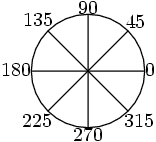arminstraub.com

# Drawing Labels

## SimplyDraw - Drawing LabelsSimply Draw introduces `label()` which can be used either globally or as a method of a canvas object.

It takes coordinates, a text (or some canvas) and optionally an angle and a distance via labelsep. Just take a look...

```set(scale=2)
stroke(circle(0, 0, 1))
for a in range(0, 360, 45):
label(0, 0, a, angle=a, labelsep=1)
stroke(line(0, 0, cos(pi/180*a), sin(pi/180*a)))
```